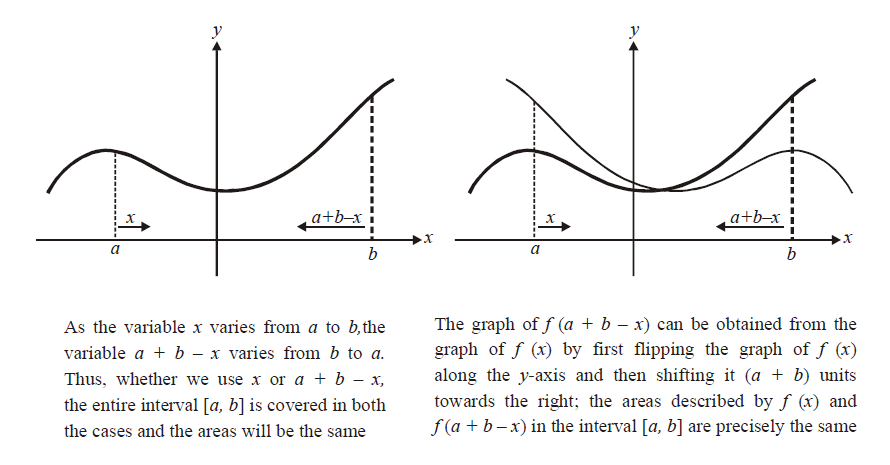# General Case of Modifying Argument of Integrand Using Limits

Go back to  'Definite Integration'

(10) We can now extend property -9 into a more general property:

$\int\limits_a^b {f(x)\,dx = \int\limits_a^b {f(a + b - x)\,dx} }$

Graphically, the justification for this property is analogous to that for property -9There is a straight forward analytical justification also. Use $$a + b - x = t$$ in the right side integral for that purpose. Property -10 is one of the most widely used properties to simplify definite integrals.

Example –16

Evaluate $$I = \int\limits_{\pi /6}^{\pi /3} {\frac{1}{{1 + \sqrt {\tan x} }}dx}$$

Solution: \begin{align}&I = \int\limits_{\pi /6}^{\pi /3} {\frac{1}{{1 + \frac{{\sqrt {\sin x} }}{{\sqrt {\cos x} }}}}dx} \\\,\, &\;= \int\limits_{\pi /6}^{\pi /3} {\frac{{\sqrt {\cos x} }}{{\sqrt {\sin x} + \sqrt {\cos x} }}\,dx} \,\,\,\,\,\,\,\,\,\,\,\,\,\,\,\,\,\,\,\,\,\,\,\,\,\,\,\,\,\,...\left( 1 \right)\end{align}

We now use property -10; we substitute \begin{align}x \to \left( {\frac{\pi }{6} + \frac{\pi }{3} - x} \right) i.e. \left( {\frac{\pi }{2} - x} \right)\end{align} in the function to be integrated. Thus,  I becomes:

$I = \int\limits_{\pi /6}^{\pi /3} {\frac{{\sqrt {\sin x} }}{{\sqrt {\sin x} + \sqrt {\cos x} }}\,dx} \,\,\,\,\,\,\,\,\,\,\,\,\,\,\,\,\,\,\,\,\,\,\,\,\,\,...\left( 2 \right)$

Adding (1) and (2), we obtain

\begin{align}&2I = \int\limits_{\pi /6}^{\pi /3} {\frac{{\sqrt {\sin x} + \sqrt {\cos x} }}{{\sqrt {\sin x} + \sqrt {\cos x} }}\,dx} \\\,\,\,\,\, &\;\;\;= \int\limits_{\pi /6}^{\pi /3} {\,dx} \\\,\,\,\, &\;\;\;= \frac{\pi }{6}\\\Rightarrow\qquad & I = \frac{\pi }{{12}}\end{align}